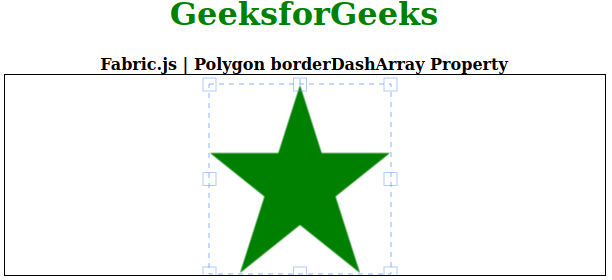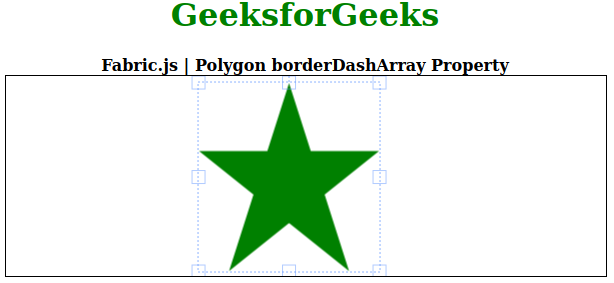Open In App

# Fabric.js Polygon borderDashArray Property

In this article, we are going to see how to set the dash pattern of the border of the canvas polygon using FabricJS. The canvas polygon means the polygon is movable and can be stretched according to requirement. Further, the polygon can be customized when it comes to initial stroke color, height, width, fill color, or stroke width.

To make it possible we are going to use a JavaScript library called FabricJS. After importing the library, we will create a canvas block in the body tag that will contain the polygon. After this, we will initialize instances of Canvas and polygon provided by FabricJS and set the dash pattern of the border of the canvas polygon using borderDashArray property and render the Triangle on the Canvas as given in the below example.

Syntax:

```fabric.Polygon([
{ x: pixel, y: pixel },
{ x: pixel, y: pixel },
{ x: pixel, y: pixel},
{ x: pixel, y: pixel},
{ x: pixel, y: pixel }],
{
borderDashArray: Array
});
```

Parameter: This property accepts a single parameter as mentioned above and described below:

• borderDashArray: This parameter defines the dash pattern of the border.

Note: Giving correct dimension pixels is a must to create a polygon.

Below examples illustrate the Fabric.JS Polygon borderDashArray property in JavaScript:

Example 1:

## HTML

 ```<``html``>`` ` `<``head``>``    ````    ``<``script` `src``=``"https://cdnjs.cloudflare.com/ajax/libs/fabric.js/3.6.2/fabric.min.js"``>``    `````` ` `<``body``>``    ``<``div` `style``=``"text-align: center;width: 600px;"``>``        ``<``h1` `style``=``"color: green;"``>``            ``GeeksforGeeks``        ````        ``<``b``>``            ``Fabric.js | Polygon borderDashArray Property``        ````    ````    ``<``canvas` `id``=``"canvas"` `width``=``"600"` `height``=``"200"` `        ``style``=``"border:1px solid #000000;"``>``    ```` ` `    ``<``script``>`` ` `        ``// Initiate a Canvas instance ``        ``var canvas = new fabric.Canvas("canvas");`` ` `        ``// Initiate a polygon instance ``        ``var polygon = new fabric.Polygon([``            ``{ x: 295, y: 10 },``            ``{ x: 235, y: 198 },``            ``{ x: 385, y: 78 },``            ``{ x: 205, y: 78 },``            ``{ x: 355, y: 198 }], {``            ``fill: 'green',``            ``borderDashArray: ``        ``});`` ` `        ``// Render the polygon in canvas ``        ``canvas.add(polygon); ``    `````` ` ``

Output:Example 2:

## HTML

 ```<``html``>`` ` `<``head``>``    ````    ``<``script` `src``=``"https://cdnjs.cloudflare.com/ajax/libs/fabric.js/3.6.2/fabric.min.js"``>``    `````` ` `<``body``>``    ``<``div` `style``=``"text-align: center;width: 600px;"``>``        ``<``h1` `style``=``"color: green;"``>``            ``GeeksforGeeks``        ````        ``<``b``>``            ``Fabric.js | Polygon borderDashArray Property``        ````    ````    ``<``canvas` `id``=``"canvas"` `width``=``"600"` `height``=``"200"` `        ``style``=``"border:1px solid #000000;"``>``    ```` ` `    ``<``script``>`` ` `        ``// Initiate a Canvas instance ``        ``var canvas = new fabric.Canvas("canvas");`` ` `        ``// Initiate a polygon instance ``        ``var polygon = new fabric.Polygon([``            ``{ x: 295, y: 10 },``            ``{ x: 235, y: 198 },``            ``{ x: 385, y: 78 },``            ``{ x: 205, y: 78 },``            ``{ x: 355, y: 198 }], {``            ``fill: 'green',``            ``borderDashArray: ``        ``});`` ` `        ``// Render the polygon in canvas ``        ``canvas.add(polygon); ``    `````` ` ``

Output: